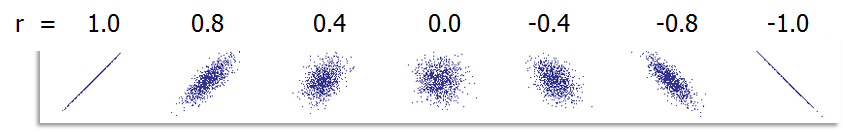# Tag Archives | coefficient correlation## Correlation Coefficient with SigmaXL

Pearson’s Correlation Coefficient Pearson’s correlation coefficient is also called Pearson’s r or coefficient of correlation and Pearson’s product moment correlation coefficient (r), where r is a statistic measuring the linear relationship between two variables. What is Correlation? Correlation is a statistical technique that describes whether and how strongly two or more variables are related. Correlation […]